• Matlab二维绘图

2021-01-16 15:59:40
二维绘图 01 plot绘图 调用格式为： 绘制一条以x为横坐标，y为纵坐标的曲线。 plot(x,y) 在同一坐标下绘制多条曲线 plot(x1,y1,x2,y2,...) 绘制一条以x为横坐标，y为纵坐标的曲线。'S'表示此曲线的类型（颜色、...

二维绘图

01 plot绘图

调用格式为：

绘制一条以x为横坐标，y为纵坐标的曲线。
plot(x,y)

在同一坐标下绘制多条曲线
plot(x1,y1,x2,y2,...)

绘制一条以x为横坐标，y为纵坐标的曲线。'S'表示此曲线的类型（颜色、线型等）
plot(x,y,'s')实践

1. 在[0 , 2 π \pi ]上，分别用星号和实线绘制f(x)=sinx和f(x)=cosx的曲线
x=linspace(0,2*pi,100);
y=sin(x);
z=cos(x);
plot(x,y,'*',x,z,'-');

运行结果：02 fplot绘图

调用格式为：

绘制自定义函数fun在区间[a,b]上的图像
fplot('fun',[a,b])

绘制自定义函数组[f1,f2,...]在区间[a,b]上的图像
fplot('[f1,f2,...]',[a,b])

实践

1. 用fplot绘制f(x)=cos(tan π \pi x x )的曲线
fplot('cos(tan(pi*x))',[0.2 0.8]);

03 ezplot绘图

绘制-2 π \pi <x<2 π \pi 上的f=f(x)的图像

ezplot(f)

绘制a<x<b上的f=f(x)的图像

ezplot('f(x)',[a,b])

绘制-2 π \pi <x<2 π \pi 和-2 π \pi <y<2 π \pi 上的f(x,y)=0的图像

ezplot('f(x,y)')

绘制x1<x<s2和y1<y<y2上的f(x,y)=0的图像

ezplot('f(x,y)',[x1,x2,y1,y2])

绘制0<t<2 π \pi 上的x=x(t),y=y(t)的参数方程图像

ezplot('x(t)','y(t)')

绘制t1<t<t2上的x=x(t),y=y(t)的参数方程图像

ezplot('x(t)','y(t)',[t1,t2])

实践

1. 绘制 x 3 + y 3 − 5 x y = 0 x^3+y^3-5xy=0 的图像
ezplot('x^3+y^3-5*x*y=0')

运行结果1. 绘制x=2cost, y=sint的图像
ezplot('2*cos(t)','sin(t)');

运行结果04 特殊的二维作图

下表为matlab的特殊二维绘图函数实践

1. 在区间[0,1]分别绘制y=sinx的条形图、阶梯图、针状图和散点图。
x=0:0.1:1;
y=sin(x);
bar(x,y)
pause       %程序暂停、任意键继续
stairs(x,y)
pause
stem(x,y)
pause
scatter(x,y)

运行结果：展开全文matlab
• matlab二维绘图

2013-03-30 23:36:39
有关Matlab二维绘图方法的讲述，函数的使用，基本的绘图方式等。

实例1----实现MATLAB程序代码如下：

>>image(X) %调出图片

>>title('大佬合照！------图1-1') %给图形命名标题

运行程序，效果如图1-1所示。

更改图形窗口的色图，并刷新图形显示，在命令窗口输入以下内容：

>>image(X)

>>colormap(gray) %内置色图函数

>>title('大佬合照！-------图1-2') %给图形命名标题

运行程序，效果如图1-2.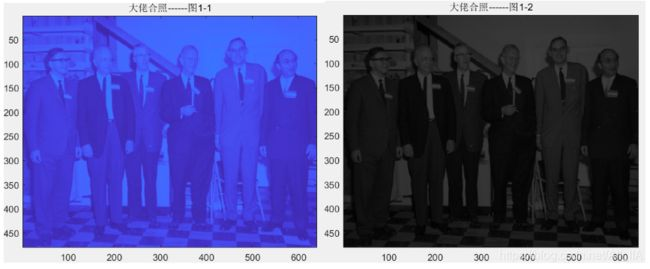例2，画正弦函数图形。MATLAB程序如下。

>>clear all; %清除命令行当前所有内容

>>x=0:pi/10:pi; %变量x的定义域参数[0,pi]

>>y=sin(x); %调用内置sin函数和plot绘图函数

>>plot(x,y); %绘图

>>grid on %给图像添加表格

运行程序，如图所示。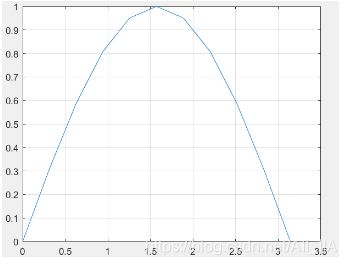MATLAB二维图形绘制

1.基本形式：

matlab 最基本的绘图函数为plot，如y是一个n维向量，那么plot(y)绘制一个y元素和y元素排列序号1，2，…，n之间的关系线性坐标图。

例如：

>>y=[1 2 3 4 2 1 0 4 9];

>>plot(y)

2.多线条形式：

调用格式：plot(X1,Y1,X2,Y2)

>>x=0:0.12:2*pi %x取[0,2pi]

>>plot(x,cos(x),x,sin(x),x,sin(2*x)) %正弦函数，余弦函数

>>grid on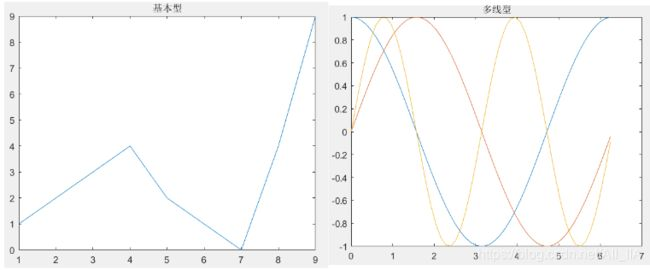图形的修改及文本标注

plot(x1,y1,选项2，x2,y2,选项2，…，xn,yn, 选项n)

其中，x1,x2…xn为x轴变量，y1,y2,…yn为y轴变量。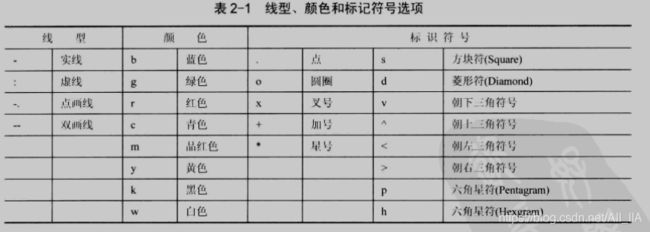例如：

>x=-pi:pi/10:pi;

>>y=tan(sin(x))-sin(tan(x));

>>plot(x,y,'--rs','LineWidth',2,'MarkerEdgeColor','k','MarkerFaceColor','g') %图形属性，’--rs‘表示虚线

>>title('plot example'); %图形标注

>>xlabel('this is x axis'); %x轴的标注

>>ylabel('this is y axis'); %y轴的标注

>>grid on %增加网格线

运行后如图所示：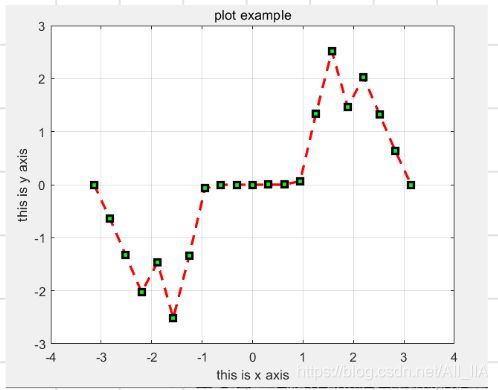图形控制

subplot(m,n,p) % mxn分布窗口，m行n列

polar(theta,rho,选项) %theta,rho分别为长度相同的角度向量和幅值向量，“选项”同plot函数

semilogx(x,y,选项) %绘制x轴为对数标度的图形

semilogy(x,y,选项)%绘制x轴为对数标度的图形

loglog(x,y,选项) %绘制两个轴均对数标度的图形

将一个图形窗口分割的行列数：p表示每一部分的代号。例如要将窗口分为4x3个部分，则左上角代号为1，右下角代号为12，MATLAB最多允许9x9个窗口的分割。

例如：特殊曲线绘制

>>clear all; %清除当前命令行

>>x=-1:0.1:1; %变量x的取值

>>subplot(2,2,1); %2x2窗口，左上角第一个

>>polar(x,exp(x)); %exp(X)，指数函数

>>subplot(2,2,2); %右上角

>>semilogx(x,exp(x)); %对数标注x坐标

>>subplot(2,2,3); %左下角

>>semilogy(x,exp(x)); %对数标度y坐标

>>subplot(2,2,4); %右下角

>>loglog(x,exp(x)) %对数标度x,y坐标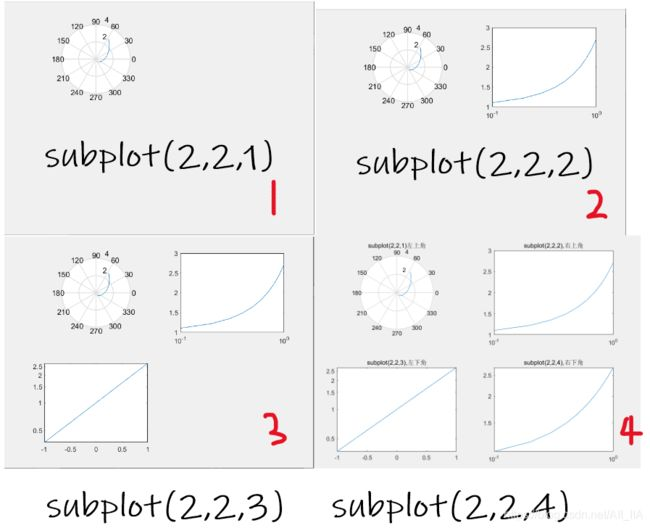展开全文• MATLAB二维绘图

2020-02-17 22:55:54
MATLAB绘图基础 1 MATLAB二维绘图 1.1 xy平面基本绘图命令 画多条曲线图 分割图形窗口 1.2 绘制图形的辅助操作 线型、标记符、颜色 线宽、标记点、字体等属性 图形设置 坐标轴设置 图形对象的修改 1.3 其他二维...

1 MATLAB的二维绘图

1.1 xy平面基本绘图命令

plotx轴和y轴均为线性刻度
loglogx轴和y轴均为对数刻度
semilogxx轴为对数刻度，y轴为线性刻度
semilogyx轴为线性刻度，y轴为对数刻度
figure生成新的图形窗口

例子：

xd = [1, 5, 50];
yd = [30, 5, 5];
loglog(xd, yd, 'blacko-', 'MarkerFaceColor', [1, 0, 0]);
axis([10^0 10^2 10^0 10^2]);
grid on;axis equal

画多条曲线图

x=0:0.1:2*pi;
plot(x,sin(x),'ro',x,cos(x),'g*');分割图形窗口

调用格式为：subplot(n,m,k)subplot(nmk)
n,m分别表示将窗口分割的行数和列数，k表示要画图部分的代号，表示第几个图形，nmk三个数可以连写,中间不用符号分开。
特殊情况：subplot(n,m,[a,b])

1.2 绘制图形的辅助操作

线型、标记符、颜色

线型说明标记符说明颜色说明
-实线（默认）+加号符r红色
- -双划线o空心圆g绿色
虚线*星号b蓝色
-.点划线.实心圆c青绿色
x叉号符m洋红色
s正方形y黄色
d菱形k黑色
^上三角形w白色
v下三角形
<左三角形
>右三角形
p五角星
h六边形

线宽、标记点、字体等属性

LineWidth线宽数值，如0.5，1等，单位为points
MarkerEdgeColor标记点边框线条颜色颜色字符，如’g’, ’b’等
MarkerFaceColor标记点内部区域填充颜色颜色字符
MarkerSize标记点大小数值
FontName字体名称
FontSize字号大小
FontAngle字体角度‘normal’–正常；‘italic’–斜体；‘oblique’–倾斜;
FontWeight字体的轻重‘light’–减轻；‘normal’–正常；‘bold’–加粗
Box属性决定图形坐标轴是否为方框形式‘on’(有方框)；‘off’(无方框)
'ColorOrder’属性设置多条曲线的颜色顺序默认值为：[1 1 0;1 0 1;0 1 1;1 0 0;0 1 0;0 0 1]黄色 粉色 天蓝 红色 绿色 兰色，还有：[1 1 1]–白色；[0 0 0]–黑色
坐标轴方向属性‘Xdir’，‘Ydir’，‘Zdir’‘normal’–正常；‘reverse’–反向
坐标轴颜色属性‘Xcolor’，‘Ycolor’，‘Zcolor’颜色字符
坐标轴网格属性‘Xgrid’，‘Ygrid’，‘Zgrid’‘on’，‘off’
坐标轴的标尺属性‘Xtick’，‘Ytick’，‘Ztick’标度的位置,值为向量
‘Xticklabel’,‘Yticklabel’,‘Zticklabel’轴上标度的符号,值与标度位置向量个数相同

图形设置

❥图形保持

hold on;

❥图形的网格

grid on %显示格线
grid off %关闭格线
grid %在两种状态之间切换

❥图形的图例

legend('y = sin(x)','y = cos(x)',……,pos);

pos指定图例框显示的位置：0–取最佳位置；1–右上角(缺省值)；2–左上角；3–左下角；4–右 下角；-1–图的右侧。

❥图形的说明文字

A.text函数：按指定位置在坐标系中写出说明文字

text(x1,y1,'字符串','选项');

选项’决定x1,y1的坐标单位，如没有选项，则x1,y1的坐标单位和图中一致。

B.gtext函数：按照鼠标点按位置写出说明文字

gtext('字符串');

坐标轴设置

❥坐标轴的控制

axis off %隐去坐标轴
axis on %显示坐标轴

❥坐标轴的说明

xlabel('Input Value'); %x轴注解
ylabel('Function Value'); %y轴注解

❥坐标轴的标题

title('Two Trigonometric Functions'); %标题

❥坐标轴刻度设置

set(gca,’XTick’,[0 1 2]);  %X坐标轴刻度数据点位置
set(gca,'XTickLabel',{'a','b','c'});  %X坐标轴刻度处显示的字符

❥坐标轴范围设置

axis([xmin xmax ymin ymax]); %设置坐标轴在指定的区间
axis auto %使用默认设置
axis manual %冻结当前坐标轴范围，以后叠加绘图都在当前坐标轴范围内显示
axis tight %采用紧密模式设置当前坐标轴范围

❥坐标轴比例设置

axis equal %等比例坐标轴
axis square %将坐标轴区域调整为正方形
axis normal %自动调整纵横轴比例，使当前坐标轴范围内的图形显示达到最佳效果

范围选项和比例设置可以联合使用，默认的设置为axis auto normal

❥坐标轴边框设置

box on; %显示边框
box off; %关闭边框
box; %切换状态

❥坐标轴位置设置
x轴位置：

set(gca,'XAxisLocation','bottom'); %将x轴的位置设置在底部(默认)。
set(gca,'XAxisLocation','top'); %将x轴的位置设置在顶部。
set(gca,'XAxisLocation','origin'); %将x轴的位置设置在y=0处。

y轴位置：

set(gca,'YAxisLocation','left'); %将y轴的位置设置在左边(默认)。
set(gca,'YAxisLocation','right'); %将y轴的位置设置在右边。
set(gca,'YAxisLocation','origin'); %将y轴的位置设置在x=0处。

❥坐标轴方向设置
x轴方向：

set(gca,'XDir','normal'); %将x轴方向设置为普通(从左到右递增)。
set(gca,'XDir','reverse'); %将x轴方向设置为反向(从右到左递增)。

y轴方向：

set(gca,'YDir','normal'); %将y轴方向设置为普通(从下到上递增)。
set(gca,'YDir','reverse'); %将x轴方向设置为反向(从上到下递增)。

图形对象的修改

❥set函数：用于设置句柄所指的图形对象的属性。

格式为：set(句柄, 属性名1, 属性值1, 属性名2, 属性值2, ……)
例子：

h=plot(x,y);
set(h,'Color', [1,0,0]); %将句柄所指曲线的颜色设为红色

❥get函数：获取指定句柄的图形对象指定属性的当前值。

格式为：get(句柄,‘属性名’)
执行后可返回X轴的当前颜色属性值[0,0,0](黑色）。
例子：

get(gca,'Xcolor'); %获得X轴的当前颜色属性值

❥如果没有设置句柄，则可以使用下列函数获得。

gcf：获得当前图形窗口的句柄；
gca：获得当前坐标轴对象的句柄；
gco：获得当前对象的句柄。
例子：

t=-pi:pi/20:pi;
y=sin(t);
plot(t,y);
set(gca,'xtick',[-pi:pi/2:pi],'xticklabel',['-pi','-pi/2','0','pi/2','pi']); %设置坐标轴的显示刻度
set(gcf, 'Color', [1,1,1]); %将图形窗口底色设为白色
set(gca, 'Xcolor', [0,1,0]); %X轴的颜色设为绿色

例子：设置坐标轴字体名称，大小，角度，轻重的同时，也会设置坐标轴注解的字体；之后可以单独设置Y轴注解的字体名称，大小。

set(gca,'FontName','Times New Roman','FontSize',14,'FontWeight','bold','FontAngle','italic')
ylabel('前角(°)','FontName','宋体','FontSize',10.508)

对字体的设置也可以用在title，xlabel，ylabel等中。
例子：

a=linspace(1,2,10);
plot(a,'--pr','linewidth',1.5,'MarkerEdgeColor','r','MarkerFaceColor','m','MarkerSize',10);
legend('a','Location','best');
title('a','FontName','Times New Roman','FontWeight','Bold','FontSize',16);
xlabel('T','FontName','Times New Roman','FontSize',14);
ylabel('a','FontName','Times New Roman','FontSize',14,'Rotation',0);
axis auto equal
set(gca,'FontName','Times New Roman','FontSize',14);

1.3 其他二维绘图函数

函数说明
bar长条图
errorbar图形加上误差范围
fplot较精确的函数图形
polar极座标图
hist累计图
rose极座标累计图
stairs阶梯图
stem针状图
fill实心图
feather羽毛图
compass罗盘图
quiver向量场图
pie饼图

❥长条图bar

bar绘制二维竖直直方图
bar3绘制三维竖直直方图
barh绘制二维水平直方图
bar3h绘制三维水平直方图

调用格式为：

bar(x,y); 或 bar(y); %默认条形类型为分组
bar(x,y,width); 或 bar(y,width); %指定每个直方条的宽度，如width>1，则直方条会重叠，默认值为width=0.8
bar(…,'grouped'); %使同一组直方条紧紧靠在一起
bar(…,'stack'); %把同一组数据描述在一个直方条上

例子：

x=1:10;
y=rand(size(x));
subplot(221);bar(x,y);%默认条形类型为分组
y=[9 8 6;2 5 8;6 2 9;5 8 7;9 4 2];
subplot(222);bar(y);%竖直直方图
subplot(223);barh(y);%水平直方图
subplot(224);bar(y,'stack');%'stack'制定条形类型为叠加
colormap(cool);%将当前颜色映象色调改为cool❥图形加上误差范围errorbar

调用格式为：

errorbar(B,X); %X为误差
errorbar(A,B,X); %X为误差
errorbar(A,B,X,Y); %X为负向误差，Y为正向误差

例子：

T=1:1:6;  %月份
A=[12,11,7,7,6,5];  %A地各月的平均值
V=[0.5,0.4,0.3,1,0.3,0.5];  %A地各月的方差值
A2=[10,8,5,4,3,3];  %B地各月的平均值
V2=[0.4,0.3,0.4,0.6,0.3,0.5];    %B地各月的方差值
subplot(121);errorbar(T,A,V,'r-o');
hold on;errorbar(T,A2,V2,'b-s');  %注意'-o'中的‘-’去掉后画出来的图是各个孤立的点
xlabel('月份');ylabel('降雨量/cm');
Vp=[0.3,0.6,0.5,0.5,0.3,0.2]; %A地各月的正向方差
Vn=[0.5,0.5,0.3,1,0.5,0.4];   %A地各月的负向方差
subplot(122);errorbar(T,A,Vn,Vp,'linewidth',2);
xlabel('月份');ylabel('降雨量/cm');❥较精确的函数图形fplot

函数特点：

绘图的数据点是自适应产生的。在函数平坦处,所取数据点比较稀疏;在函数变化剧烈处,将自动取较密的数据点。

调用格式为：

fplot(fun,limits,tol,linespec);
fun:函数名,可以是MATLAB已有函数、自定义的M函数或字符串定义的函数。
limits:表示绘制图形的坐标轴取值范围,有两种方式:[xminxmax]和[xminxmaxyminymax]。
tol:相对误差,默认值为2e-3。
linespec:表示图形的线型、颜色和数据点等设置。

例子：

x=-1:0.1:1; %定义域取值范围和步长
fplot('sin(1./x)',[0.02 0.2]); %[0.02 0.2]是绘图范围❥极座标图polar

调用格式为：

polar（theta，rho，选项）; %theta为极坐标极角，rho为极径，选项的内容和plot函数相似

例子：

theta=0:0.01:2*pi;
r=cos(4*theta);
polar(theta,r,'b');❥累计图hist

对于大量的资料，我们可用hist来显示资料的分情况和统计特性。
调用格式为：

hist(x); %x是一维向量，函数的作用是将x中的最小和最大值之间的区间等分成10等份，输出的直方图横坐标是x值，纵坐标是该值的个数
[a,b]=hist(x,n); %其中x是一维向量，函数功能是将x中的最小和最大值之间的区间等分n份，横坐标是x值，纵坐标是该值的个数。返回的a是落在该区间内的个数，b是该区间的中心线位置坐标。

例子：

x=[1,2,3,4,5,6,1,2,5,4,7,8,5,6,4,6];
subplot(121),hist(x);
x=randn(200, 1); %产生5000个m=0,s=1的高斯乱数
subplot(122),hist(x,15); %20代表长条的个数❥极座标累计图rose

rose和hist很接近，只不过是将资料大小视为角度，资料个数视为距离，并用极座标绘制。
例子：

x=randn(1000, 1);
rose(x);❥阶梯图stairs

调用格式为：

stairs(Y);
stairs(X,Y);

例子：

x=linspace(-2*pi,2*pi,40);
subplot(211),stairs(x,sin(x))
hold on;plot(x,sin(x),':*');
rng('default');
n=rand(1,10);
subplot(212),stairs(n);❥针状图stem

stem(X,Y)绘制二维针状图
stem3(X,Y,Z)绘制三维针状图

调用格式为：

stem(Y); %将数据序列Y从x轴到数据值按照茎状形式画出，以圆圈终止。如果Y是一个矩阵，则将其每一列按照分隔方式画出。
stem(X,Y); %在X的指定点处画出数据序列Y
stem(...,'filled'); %以实心的方式画出茎秆
stem(...,'LINESPEC'); % 按指定的线型画出茎秆及其标记

例子：

x=0:0.2:10;
y=sin(x).*exp(-x/3);
subplot(221),stem(x,y); %绘制二维针状图
subplot(222),stem(x,y,':sr'); %改变线型、标记点形状和颜色
subplot(223),stem(x,y,'fill');%填充颜色
A=[1,2,3;4,6,7];
subplot(224),stem3(A); %绘制三维针状图❥实心图fill

fill绘制二维填充图
fill3绘制三维填充图

调用格式为：

fill(X,Y,C); %创建填充多边形顶点的颜色从C中指定的X和Y的数据是一个向量或矩阵用于作为颜色表索引。如果C是一个行向量，长度（C）必须大小相等的（x，2）和大小（Y，2）；如果C是一个列向量，长度（C）必须大小相等的（x，1）和大小（Y，1）。如果有必要，填充闭合多边形的顶点连接最后一。
fill(X,Y,ColorSpec); %二维多边形填充指定的X和Y与指定的colorspec颜色
fill(X1,Y1,C1,X2,Y2,C2,...); %指定多个二维填充区域
fill(...,'PropertyName',PropertyValue); %允许您指定一个补丁的图形对象的属性的名称和值。
h=fill(...); %返回一个向量处理图形对象的补丁，每个补丁的对象一柄
fill3(X,Y,Z,C)
fill3(X,Y,Z,ColorSpec)
fill3(X1,Y1,Z1,C1,X2,Y2,Z2,C2,...)
fill3(...,'PropertyName',PropertyValue)
fill3(ax,...)
h=fill3(...)

例子：

x=-2*pi:pi/100:2*pi;
y=sin(x);
subplot(121),fill(x,y,'g');%绘制二维填充图
axis([-7,7,-1.5,1.5])
hold on;
fill([0:pi/100:pi],sin([0:pi/100:pi]),'r');
fill([-2*pi:pi/100:-pi],sin([-2*pi:pi/100:-pi]),'r');
hold off
X=[0;1;1;0];
Y=[1;1;0;0];
Z=[1;1;0;0];
subplot(122),fill3(X,Y,Z,[0.1 0.2 0.3]);%绘制三维填充图❥羽毛图feather

调用格式为：

feather(x,y) 函数绘制由向量参量x与y构成的速度向量，沿水平轴方向，从均匀间隔点以箭头发射出来；
feather(z) 函数绘制羽毛图。参量z是一个复数，则feather(z)相当于compass(real(z)，imag(z))；
feather(...,LineSpec) 函数绘制羽毛图。参量LineSpec指定的线型、标记符号、颜色等属性画出羽毛图；
H = feather(...) 函数返回羽毛图中线型对象的句柄H。

例子：

theta=linspace(0, 2*pi, 20);
z = cos(theta)+i*sin(theta);
subplot(211),feather(z);
theta=-pi/2:pi/16:pi/2;
r=2*ones(size(theta));
[u,v]=pol2cart(theta,r);%把极坐标（柱坐标）转换为笛卡尔坐标（指平面直角坐标或空间直角坐标）。
subplot(212),feather(u,v);❥罗盘图compass

调用格式为：

compass(x,y); %函数绘制一个由原点出发由(x,y)组成的向量箭头图形；
compass(z); %等价于compass（real(z),imag(z))；
compass(...,LineSpec); %函数用参量LineSpec指定箭头的线型、标记符号、颜色等属性；
h=compass(...); %函数返回line对象的句柄给h。

例子：

theta=linspace(0,2*pi,20);
z=cos(theta)+i*sin(theta);
subplot(121),compass(z,'-.r');
x=1:100;
y=rand(1,100);
subplot(122),compass(x,y);❥向量场图quiver

调用格式为：

quiver(x,y,u,v); %用来绘制二维方向箭头，表示通过在(x, y)指定的位置绘制小箭头来表示以该点为起点的向量(u,v)。x,y,u,v必须是相同阶数。
quiver(u,v); %在x-y面上绘制向量(u,v)，由于没有指定向量的起点，所以，matlab将在x-y面上均匀的取若干个点作为起点。

例子：

u=[1 1 1; 1 1 1];v=u;
subplot(121),quiver(u, v);
x=[0 0 0 0];y=x;%指定起点为(0,0)
u=[1 0 -1 0];v=[0 1 0 -1];
subplot(122),quiver(x, y, u, v);❥饼图pie

pie(X)绘制二维饼图
pie3(X)绘制三维饼图

调用格式为：

pie(X); %利用向量X中的数据描绘饼图，X中的数据被看做频数，饼图中比率的获得：X中的元素x[i]/sum(X)。当X中所有元素的和sum（X）< 1.0时，图形不是一个整圆。
pie(X,{'...', '...', ...}); %‘...’是标注。
pie(X,y); %将一些扇区从饼图中分离出来，y为一个与X尺寸相同的矩阵，其非零元素所对应X矩阵中的元素从饼图中分离出来。

例子：

x=[5.5 74.7 44.5 33.2 46.6];
subplot(231),pie(x); %不分离饼图
subplot(232),pie(x,{'1', '2', '3','4','5'}); %‘...’是标注。
subplot(233),pie(x,[0 0 0 0 1]);%分离饼图，将第五个元素分离出来
x1=[0.2 0.3 0.4];
subplot(234),pie(x1); %不完整饼图
x2=[1 2 4 3 5];
subplot(235),pie3(x2); %绘制三维饼图
subplot(236),pie3(x2,[0 1 0 1 0]); %将第二、四个元素分离出来展开全文matlab
• MATLAB二维绘图

2020-07-17 19:28:35
MATLAB绘图篇—二维绘图 目录MATLAB绘图篇—二维绘图绘图基本函数—plot函数1.plot参数为基本参数plot函数基本调用方式plot函数其他调用方式2. 含多个输入参数的plot函数3. 含选项的plot函数4. 双纵坐标函数plotyy ...

MATLAB二维绘图

绘图基本函数—plot函数

1.plot参数为基本参数

plot函数基本调用方式

plot(x,y)
x和y为长度相同的向量，分别用于存储横坐标和纵坐标。

x=0:pi/100:2*pi;
y=2*exp(-0.5*x).*sin(2*pi*x);
plot(x,y)

%这里x，y为步长为π/100，从0到2π，长为201列的向量。
他们的列数相同，行数只有一条，因此以x作为横坐标，y对应元素作为纵坐标，
然后绘制一条曲线。

输出结果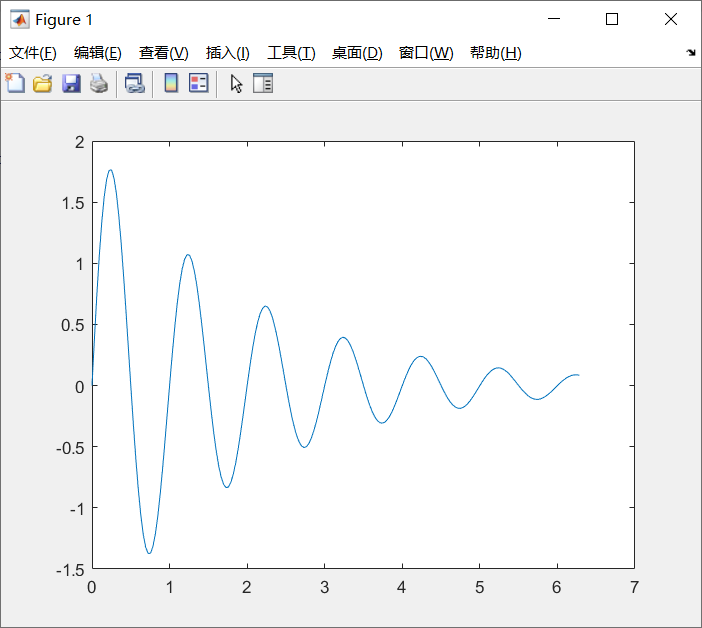plot函数其他调用方式

（1）当x是向量，y是有一维与x同维的矩阵时，则绘制出多根不同颜色的曲线。曲线条数等于y矩阵的另一维数，x被作为这些曲线共同的横坐标。

x=linspace(0,2*pi,100);
y=[sin(x);cos(x)];
plot(x,y)

%x为长度100列向量，y为2行100列的矩阵，他们列数相等，因此用y的另一维作为
条数，就是用行数作为条数，此处就有2条曲线。然后就是x的第一行第一列
元素，对y的第一行第一列元素，依次类推出第一条曲线。

输出结果为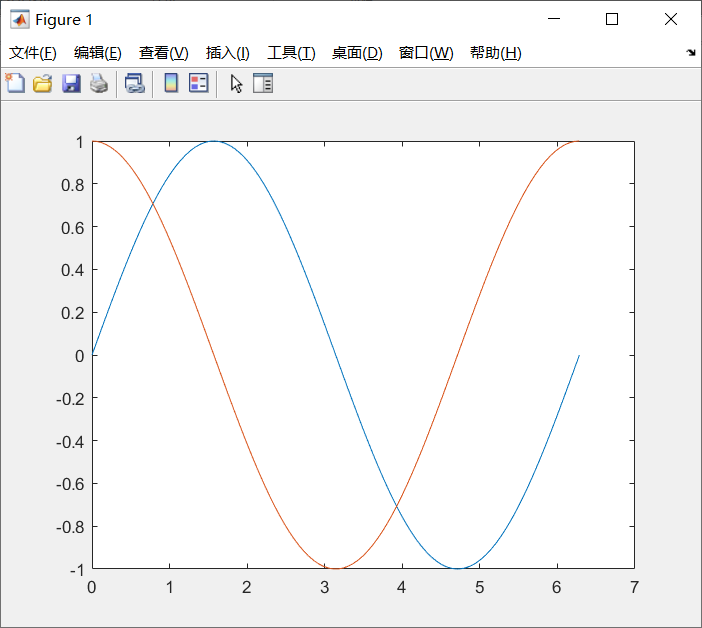（2） 当x,y是同维矩阵时，则以x,y对应列元素为横、纵坐标分别绘制曲线，曲线条数等于矩阵的列数。

t=linspace(0,2*pi,100);
x=[t;t]';
y=[sin(t);cos(t)]';
plot(x,y)

%次数t为有100个元素的行向量，x，y为100行2列的矩阵。以为维数相同，列数为
2列，因此有两条曲线。x的第一列作元素作为横坐标，对应y的第一列元素，作为
纵坐标。

输出结果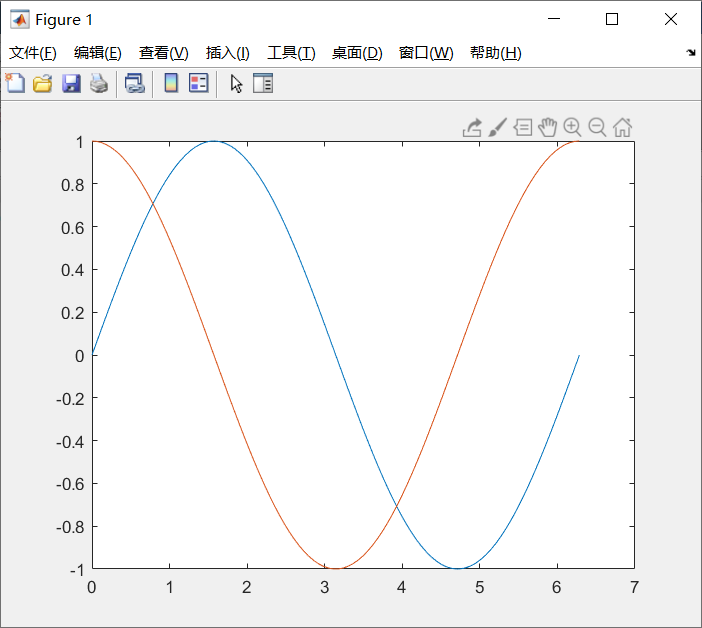（3）plot函数最简单的调用格式是只包含一个输入参数：
plot(x)
①当x是实向量时，以该向量元素的下标为横坐标，元素值为纵坐标画出一条连续曲线，这实际上是绘制折线图。

当x是复数向量时，则分别以该向量元素实部和虚部为横、纵坐标绘制出一条曲线。

%一个单位圆
t=0:0.1:2*pi;
x=exp(i*t);		%cos(t)+i*sin(t)
plot(x)
axis equal;

%这里t为长为63的行向量，x为长63个成员，成员为复数的行向量，则以每个向量
的实部作为横坐标，虚部作为纵坐标，绘制一个圆。

输出结果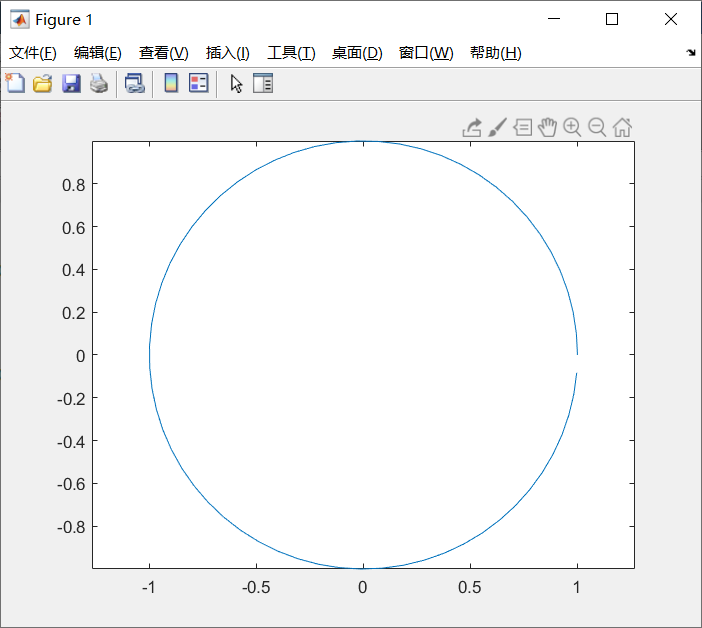②当输入参数是复数矩阵时，则按列分别以元素实部和虚部为横、纵坐标绘制多条曲线。

%三个同心圆
t=0:0.01:2*pi;
x=exp(i*t);
y=[x;2*x;3*x]';
plot(y)
axis equal;

%此处t为步长0.01，从0到2π的行向量。x为长639列的行向量，y是3行639列矩阵
的转置矩阵，为639行3列。因此有3条曲线，每条曲线以每列元素的实部作为横
坐标，虚部作为纵坐标，绘制出3条曲线。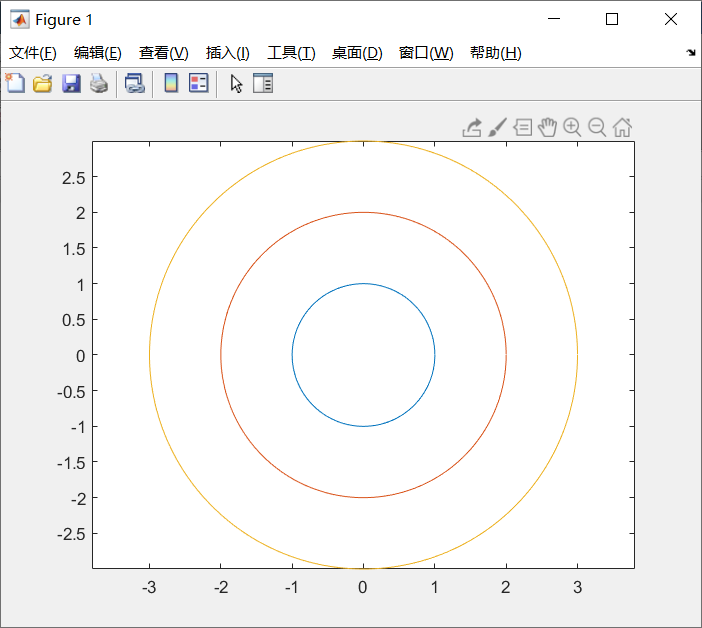③当输入参数是实矩阵时，则按列绘制每列元素值相对其下标的曲线，曲线条数等于输入参数矩阵的列数。

2. 含多个输入参数的plot函数

调用格式为：
plot(x1,y1,x2,y2,…,xn,yn)
① 当输入参数都为向量时，x1和y1，x2和y2，…，xn和yn分别组成一组向量对，每一组向量对的长度可以不同。每一向量对可以绘制出一条曲线，这样可以在同一坐标内绘制出多条曲线。
例如

x=linspace(0,2*pi,100);
plot(x,sin(x),x,2*sin(x),x,3*sin(x))

%此处为x与sin(x)匹配，x作为横坐标，sin(x)作为纵坐标，后面两条曲线的参数选取同理。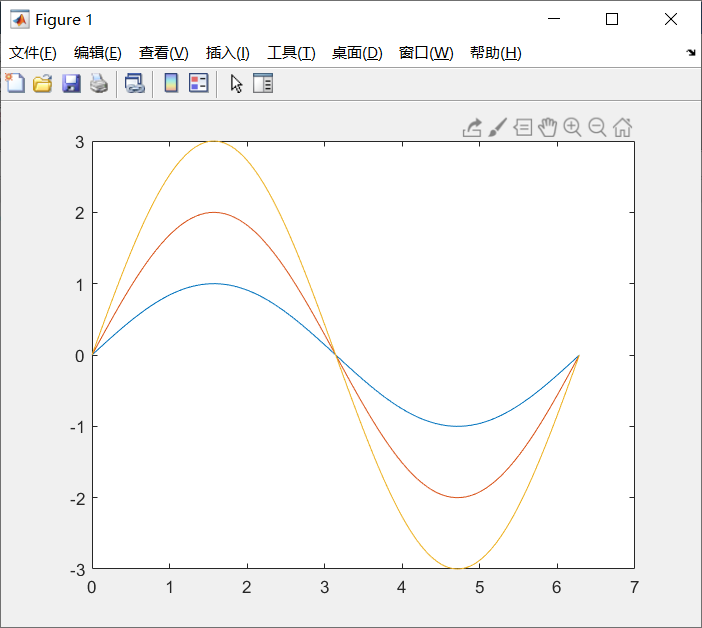②当输入参数有矩阵形式时，配对的x,y按对应列元素为横、纵坐标分别绘制曲线，曲线条数等于矩阵的列数。

x=linspace(0,2*pi,100);
y1=sin(x);
y2=2*sin(x);
y3=3*sin(x);
x=[x;x;x]';
y=[y1;y2;y3]';
plot(x,y,x,cos(x))

：此处x先是长为100的行向量，之后为转置矩阵，为100行3列，不过3列的内容都相同。y也为100行3列。plot打印出来时，是先有x矩阵的每一列元素，作为每条曲线的横坐标。然后y的每一列元素作为曲线的纵坐标，由于纵坐标不同，所有看到三条曲线。同理，第二组匹配，但是第二组的三条曲线由于横纵坐标相同，因此是重合在一次，只看到一条。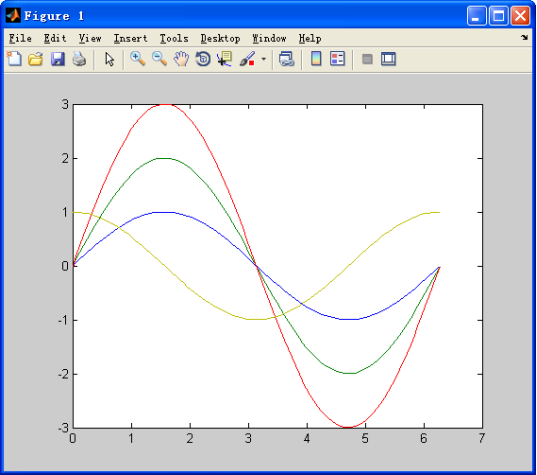3. 含选项的plot函数

绘图选项用于确定所绘曲线的线型、颜色和数据点标记符号，它们可以组合使用。
当选项省略时，线型一律用实线，颜色将根据曲线的先后顺序依次按下表给出的前7种颜色。
要设置曲线样式可以在plot函数中加绘图选项，调用格式：
plot(x1,y1,选项1,x2,y2,选项2,…,xn,yn,选项n)

表 符号选项及其含义

线型符号含义颜色符号含义标记符号含义
-实线b蓝色.
:虚线g绿色o圆圈
-.点划线r红色x叉号
双划线c青色+加号
m品红色*星号
y黄色s方块符
k黑色d菱形符
w白色v朝下三角符号
^朝上三角符号
<朝左三角符号
>朝右三角符号
p五角星符
h六角星符

注:可由help plot查询
例如

x=linspace(0,2*pi,100);
y1=sin(x);
y2=2*sin(x);
y3=3*sin(x);
x=[x;x;x]';
y=[y1;y2;y3]';
plot(x,y,'r-',x,cos(x),'m--')

输出结果为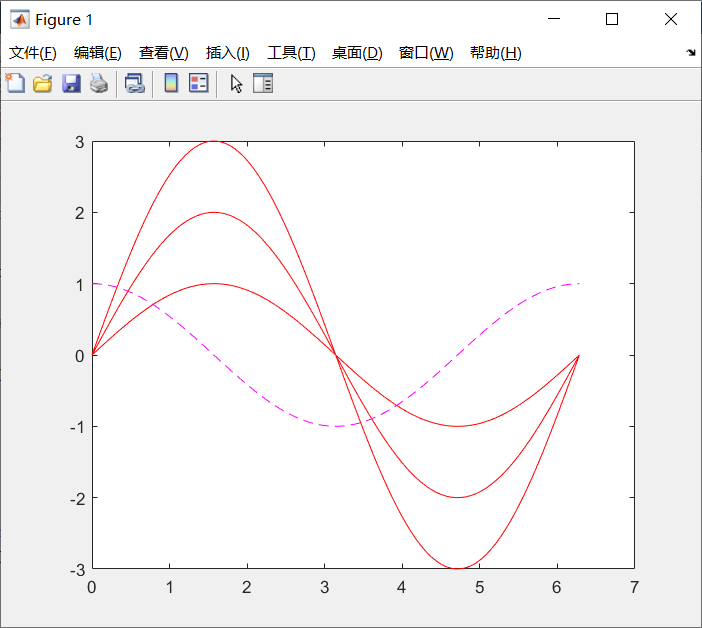4. 双纵坐标函数plotyy

绘制出具有不同纵坐标标度的两个图形的函数调用格式为：
plotyy(x1,y1,x2,y2)
x1,y1对应一条曲线，x2,y2对应另一条曲线。
左纵坐标轴用于x1,y1数据对，右纵坐标轴用于x2,y2数据对。
例如

x1=0:pi/100:2*pi;
x2=0:pi/100:3*pi;
y1=exp(-0.5*x1).*sin(2*pi*x1);
y2=1.5*exp(-0.1*x2).*sin(x2);
plotyy(x1,y1,x2,y2);

输出结果为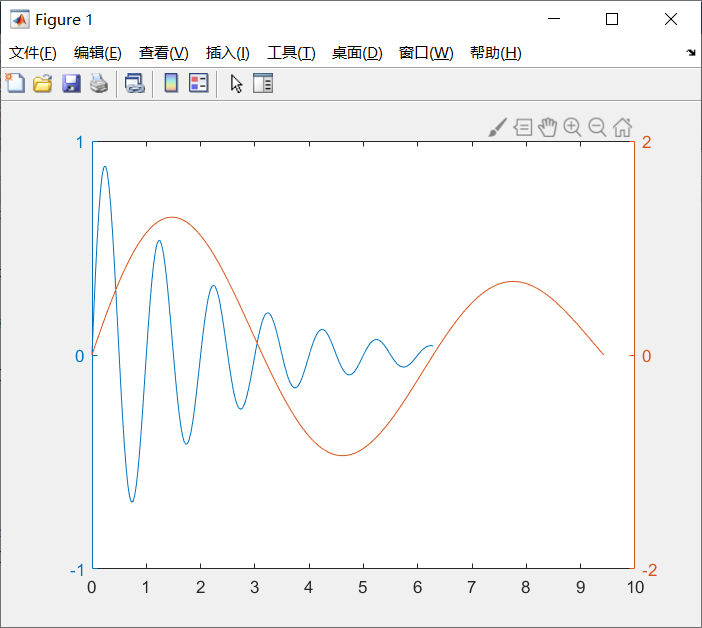可以看出左右链表的纵坐标轴不同，表示的两条曲线的数值也不同。

5.图形窗口的切割

subplot函数的调用格式为：
subplot(m,n,p)
将当前图形窗口分成m×n个绘图区，即每行n个，共m行，区号按行优先编号，且选定第p个区为当前活动区。
在每一个绘图区允许以不同的坐标系单独绘制图形（子图）。

x1=0:pi/100:2*pi;
x2=0:pi/100:3*pi;
y1=exp(-0.5*x1).*sin(2*pi*x1);
y2=1.5*exp(-0.1*x2).*sin(x2);
subplot(2,2,2);
plotyy(x1,y1,x2,y2);

%此处是将图形窗口分为四份，从左上开始按行数，第二块为活动绘图区。

输出结果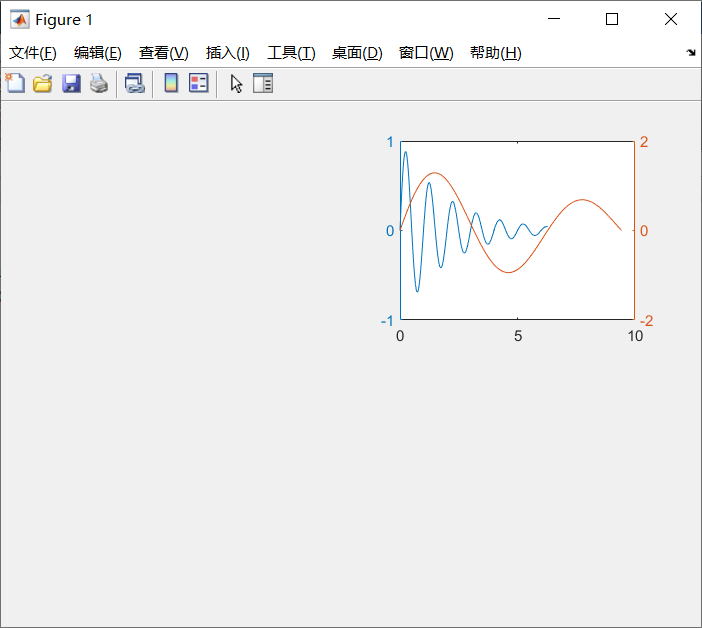展开全文matlab
• 为不常用matlab作图，但偶尔需要的Liu同学准备的，繁琐，还是比较实用的。 一、数据导入流程 1、新建脚本文件（方便重复使用） 2、导入数据 将Excel表格数据导入到数组num中，如下： (1)num=xlsread(filename...
•MATLAB
• MATLAB二维绘图(四)其他特殊的二维绘图函数介绍及用法 1、使用semilogy、semilogx和loglog绘制指数函数的图像，示例： %% 对指数进行绘图，使用不同的绘图函数 clear; clc; close all; x = logspace(-1,1,100); % ...
• Matlab二维绘图---plot函数详解

万次阅读 多人点赞 2017-09-15 21:20:59
plot函数是matlab中最主要的二维作图函数。（参考文献--MATLAB HELP 文档）   一、常用的函数主要的形式有以下几种：   plot(X,Y) plot(X,Y,LineSpec) plot(X,Y)：根据X 和 Y 绘制二维图， X,Y 必须有...matlab plot
• 完成二维曲线及其标注，任选函数完成一组二维曲线的绘图, 曲线颜色、坐标和分格线等,达到了解 MATLAB 操作基础,以及二维绘图基本操作的目的。
• MATLAB二维绘图命令画一个你认为最有趣的图形matlab
• Matlab二维绘图数据源分析及曲线族绘制.pdf
• matlab的图形绘制程序，包括二维图和三维图，较基础，非常适合初学者使用。MATLAB
• matlab二维绘图案例PPT教案学习.pptx
• matlab二维绘图剖析PPT教案学习.pptx
• 1、二维图形plotplot(x1,y1,x2,y2) —— 多条曲线绘图格式plot(x,y,’s’) —— 开关格式，开关量字符串s设定曲线颜色和绘图方式，使用颜色字符串的前1~3个字母，如yellow—yel表示等。或plot(x1,y1,’s1’,x2,y2,’...
• 需要求多个峰值的二维矩阵如下： figure(); % 总功率谱绘图 surf(MatrixA); 绘图结果： 然后求取峰值位置（需要安装图像处理工具箱）： PeaksMap = imregionalmax(MatrixA); 其得到的结果如下，可以看到是峰值的...
• matlab的二维绘图

万次阅读 多人点赞 2018-11-11 09:47:42
matlab绘制图形的步骤为： 数据准备：产生自变量采样向量，计算相应的函数值向量。 选定图形窗口及子图位置：在默认情况下，MATLAB系统绘制的图形为figure(1).figure(2),... 调用绘制函数绘制图形，例如plot函数 ...matlab 基础 笔记
• MATLAB 维图绘制;主要内容;基本函数plot;基本函数plot;基本函数plot;基本函数plot;基本函数plot;基本函数plot;图形窗口设置;图形窗口设置;图形窗口设置;图形窗口设置;标注图形;标注图形;MATLAB绘图例子;Thank you
• matlab二维绘图的一些总结

千次阅读 2017-11-29 14:15:04matlab绘图
• MATLAB二维绘图及图形设置方法 > 1、二维数据曲线图 plot函数的基本调用格式为： plot(x,y) 其中x和y为长度相同的向量，分别用于存储x坐标和y坐标数据。 例1 在0≤x≤2区间内，绘制曲线 y=2e-0.5xcos(4πx)...matlab 开发语言
• matlab实验报告-二维绘图实验报告2课程 数学实验与数学软件 实验名称 matlab二维绘图 第 1 页专业 数学与应用数学 班级_08级数学3班__ ______ 学号_182__ 姓名 郑贞贞 实验日期：2010 年 03 月18 日 评分一、实验...
• matlab二维图形的绘制 MATLA‎B技术论坛‎ www.matla‎ 账号zap‎2004 密码zap‎2004 matla‎b二维图形‎的绘制 2007年‎12月17‎日 星期一 10:37 常用的二维‎图形命令: plot：绘制二维图‎形 loglo‎g：用全对数坐...
• 颜色： 字符 b c g k m r w y 颜色 蓝色 青色 绿色 黑色 洋红 红色 白色 黄色 线型： ... 字符...plot matlab
• MATLAB二维绘图(二)向图中添加标题，坐标轴，图标和文字信息 1、添加标题、图例、x轴信息和y轴信息，示例： %% 添加标题 clear; clc; close all; x = 0:0.1:2*pi; y1 = sin(x); y2 = exp(-x); plot(x, y1, '--*',...
• matlab程序解决二维稳态热传导方程的程序，采用差分法迭代求解相关的数值解。可以有效的模拟相关平板热传导的热力场。
• 一、绘制正弦函数图像、 1、二维绘图步骤、 2、二维绘图步修饰、 3、代码示例、 二、设置图像颜色、matlab
• Matlab 二维绘图与三维绘图函数总结 参考资料：《Matlab编程及其应用第三讲》——陆伟 一. 二维绘图 1. 基本绘图函数：plot函数。 2. 功能：对 xxx 坐标及对应的 yyy 坐标绘图。 3. 使用格式： plot(x, y, ...); ...matlab
• Matlab二维绘图函数

千次阅读 2015-02-17 23:31:27
matlab绘图函数的基本使用，简要介绍了figure，subplot，hold，axis和close的简要用法。与初学者共勉，互相学习，共同进步。matlab figure subplot hold axis...

matlab二维绘图matlab 订阅